价值13000svip视频教程,前端大神匠心打造,零基础前端开发工程师视频教程全套,基础+进阶+项目实战,包含课件和源码

出租广告位,需要合作请联系站长

+关注

2021-07(7)

2021-08(64)

2021-09(79)

2021-10(103)

2021-11(94)

## 产品经理：你能不能用 DIV 给我画条龙？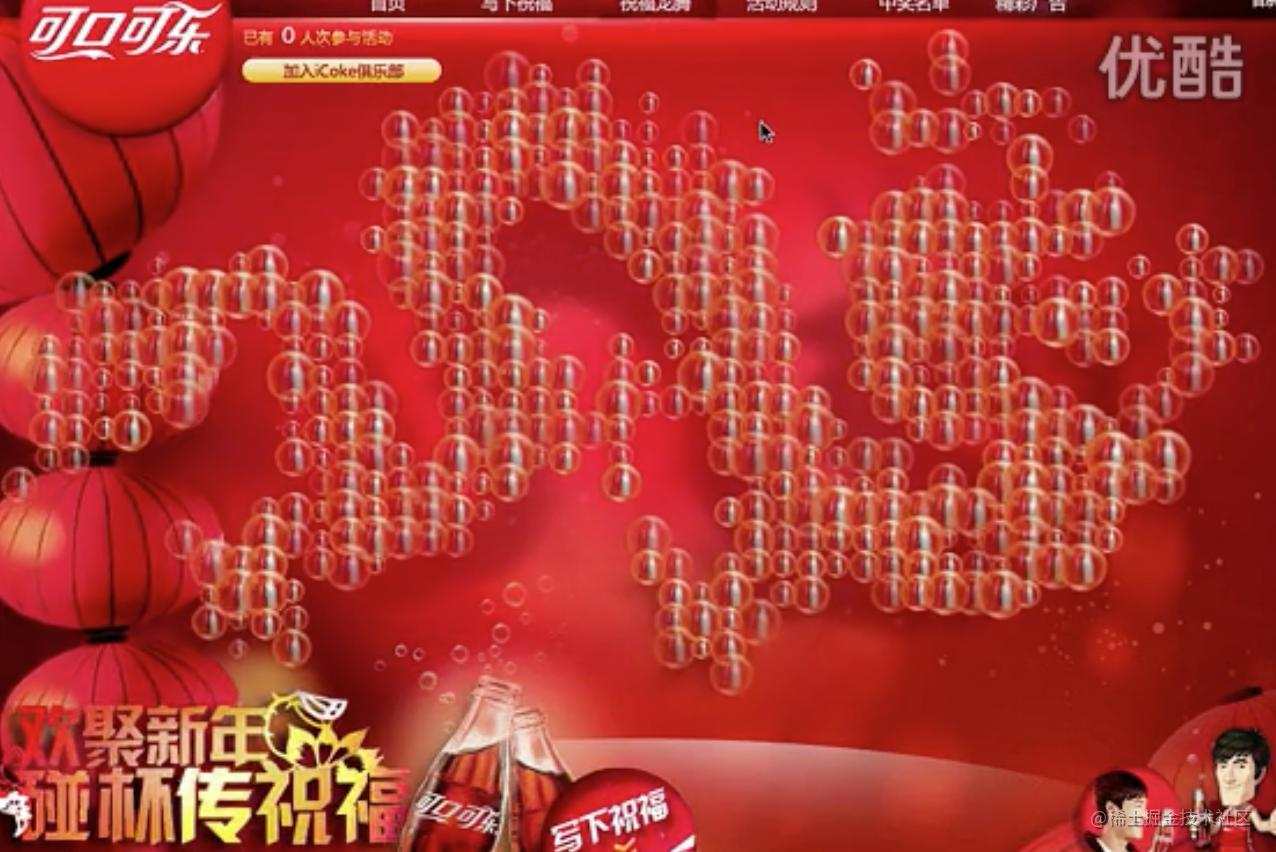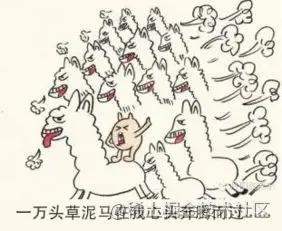# 龙从哪里来？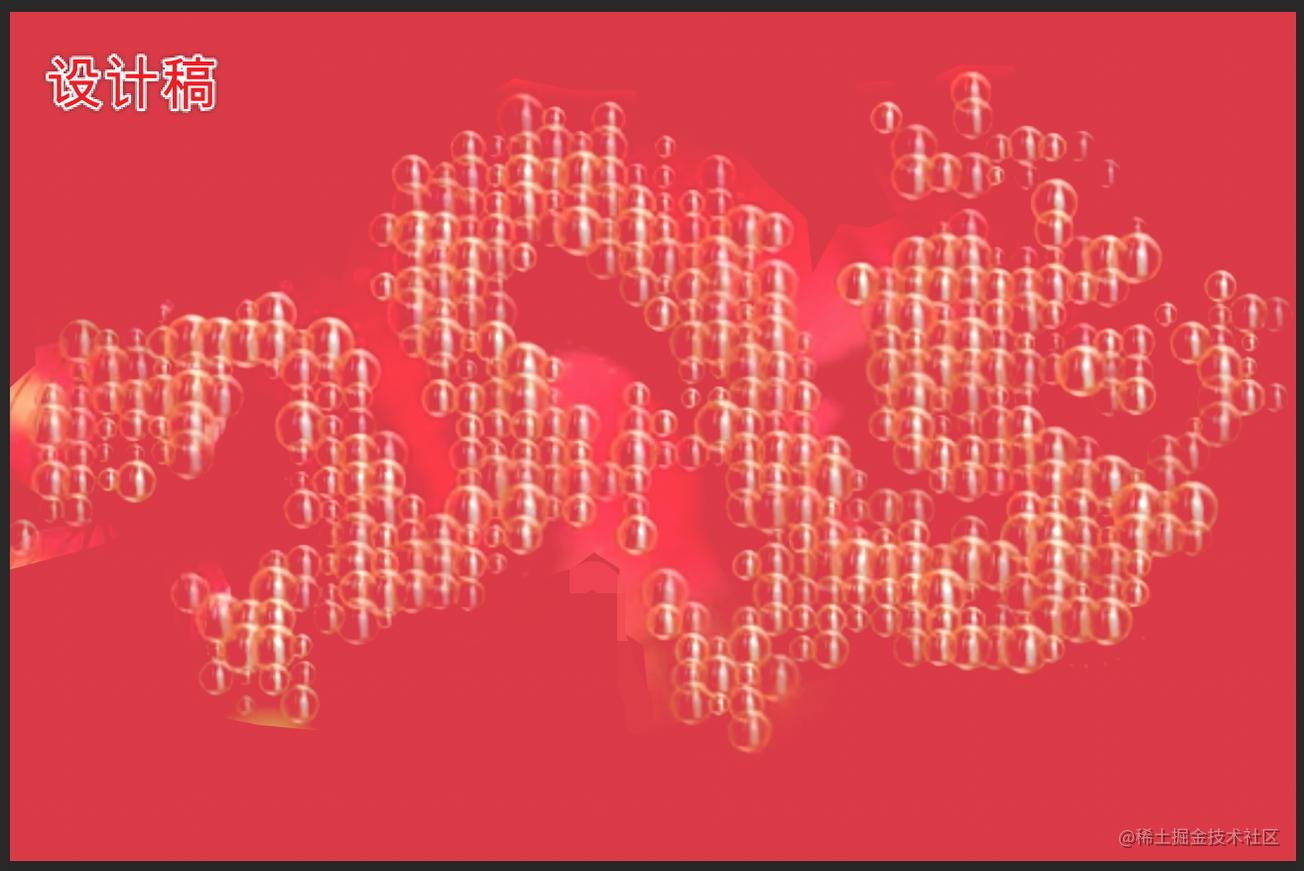# 拆解需求

• 需求1：有鼠标交互效果（太简单）
• 需求2：气泡要浮动（css动画，easy）
• 需求3：气泡组成一条龙

`诶，画个龙`

`用什么画，canvas`

`canvas能获得指定区域的像素点阵`

# 代码时间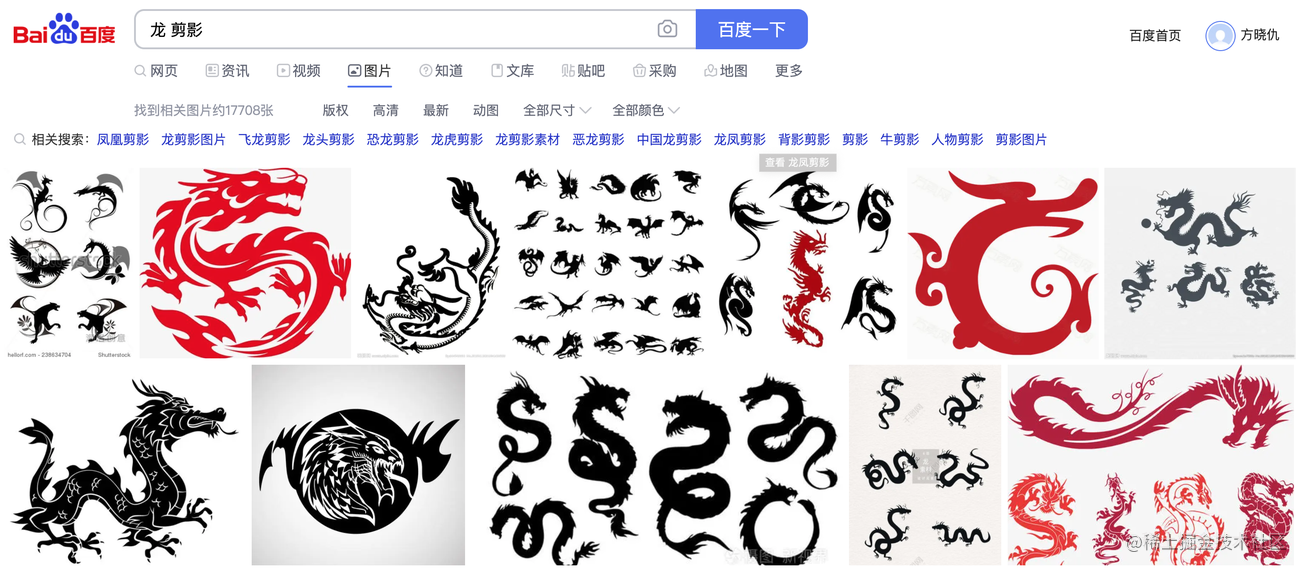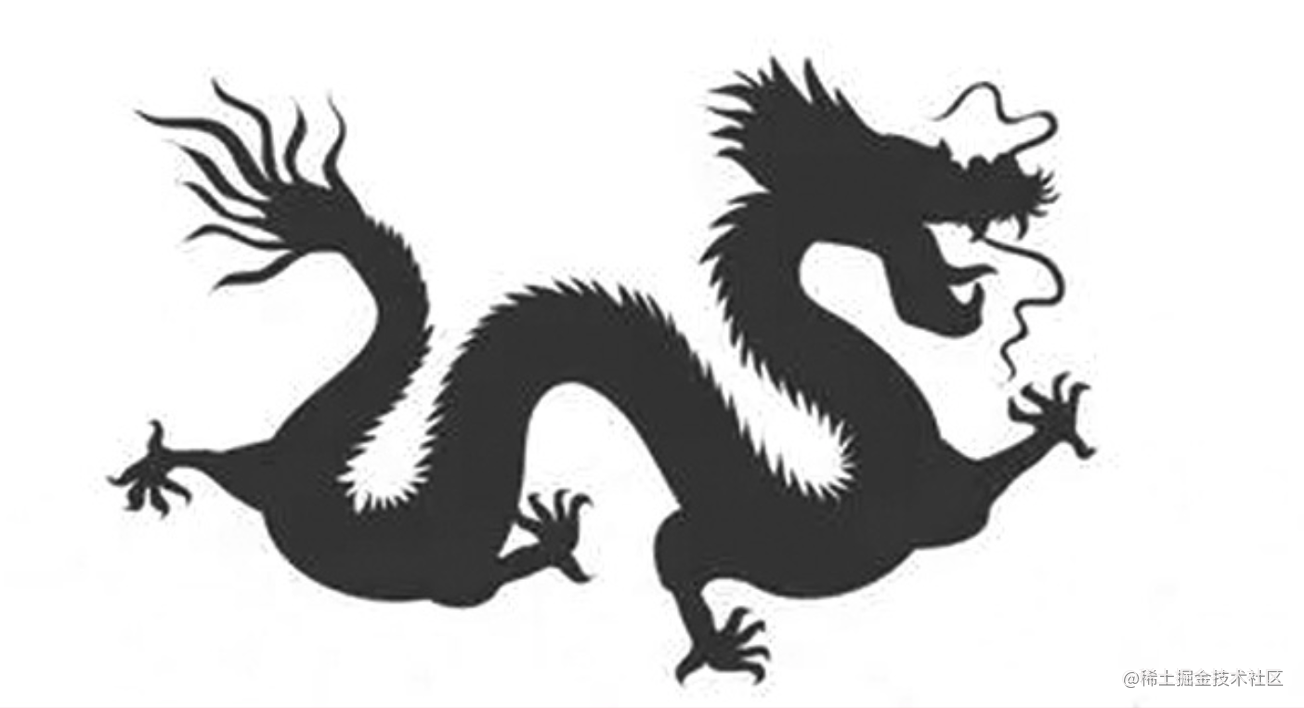``var canvas = document.getElementById("canvas");var ctx = canvas.getContext("2d"); var image = new Image();image.src = "dragon.jpg";image.onload = function(){        canvas.width = image.width;        canvas.height = image.height;         ctx.drawImage(image,0,0);}``

获取并裁剪画布的点阵信息

``var imageData = ctx.getImageData(0,0,image.width,image.height).data;ctx.fillStyle = "#ffffff";ctx.fillRect(0,0,image.width,image.height); var gap = 6; for (var h = 0; h < image.height; h+=gap) {    for(var w = 0; w < image.width; w+=gap){            var position = (image.width * h + w) * 4;            var r = imageData[position], g = imageData[position + 1], b = imageData[position + 2];             if(r+g+b==0){                    ctx.fillStyle = "#000";                    ctx.fillRect(w,h,4,4);                }    }}``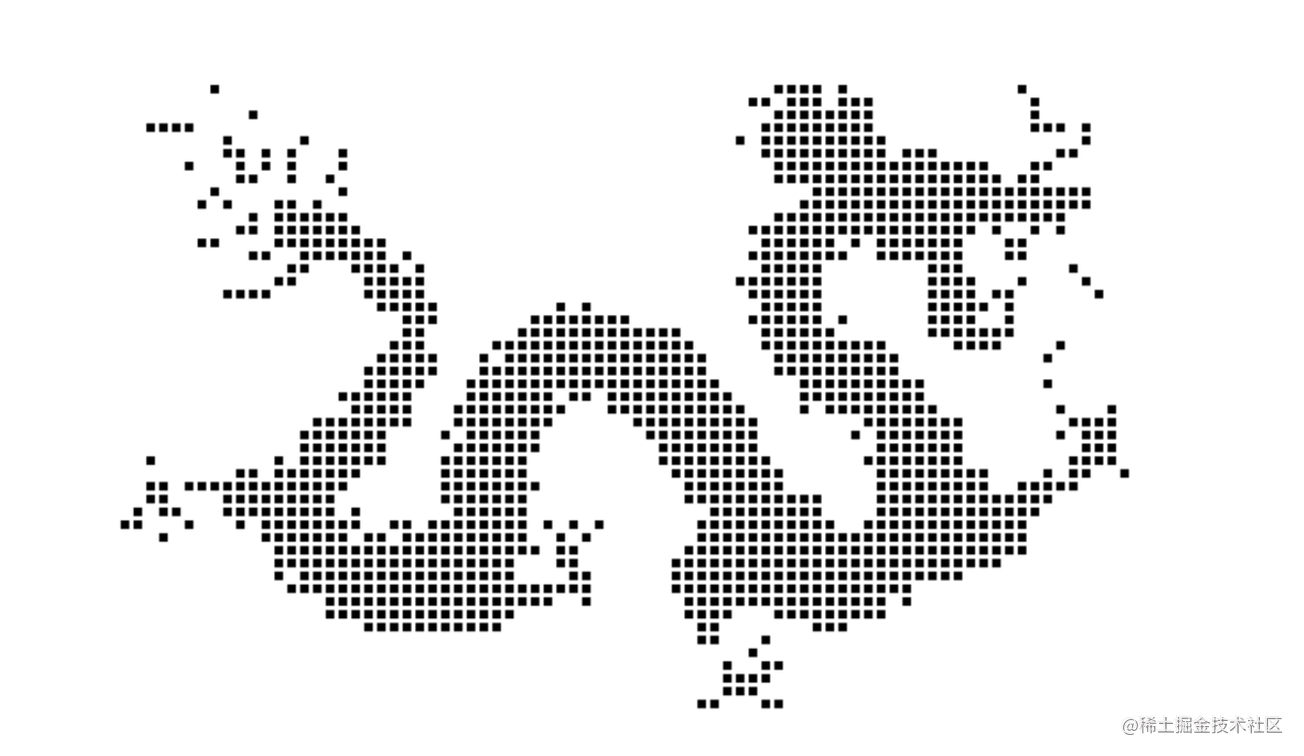``var dragonContainer = document.getElementById("container");var dragonScale = 2; for (var h = 0; h < image.height; h+=gap) {    for(var w = 0; w < image.width; w+=gap){            var position = (image.width * h + w) * 4;            var r = imageData[position], g = imageData[position + 1], b = imageData[position + 2];             if(r+g+b==0){                    var bubble = document.createElement("img");                    bubble.src = "bubble.png";                    bubble.setAttribute("class","bubble");                     var bubbleSize = Math.random()*10+20;                    bubble.style.left = (w*dragonScale-bubbleSize/2) + "px";                    bubble.style.top = (h*dragonScale-bubbleSize/2) + "px";                    bubble.style.width = bubble.style.height = bubbleSize+"px";                    bubble.style.animationDuration = Math.random()*6+4 + "s";                     dragonContainer.appendChild(bubble);                }    }}``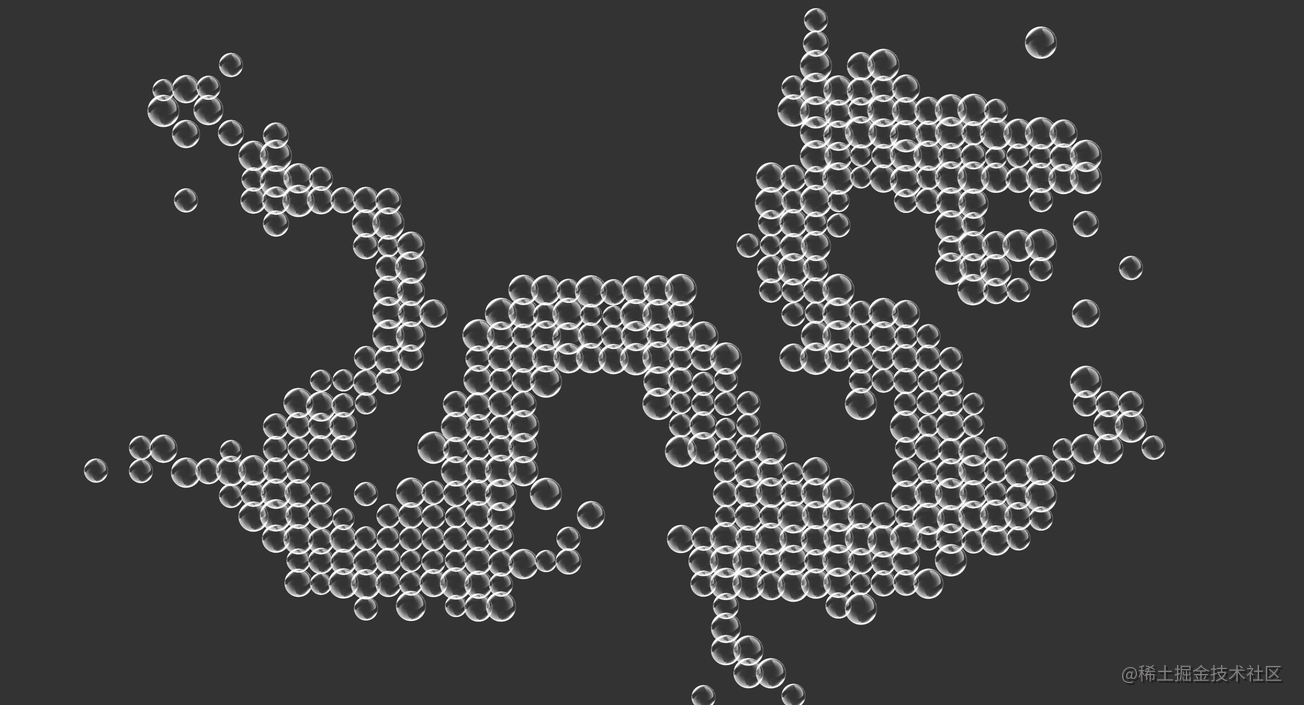11 0